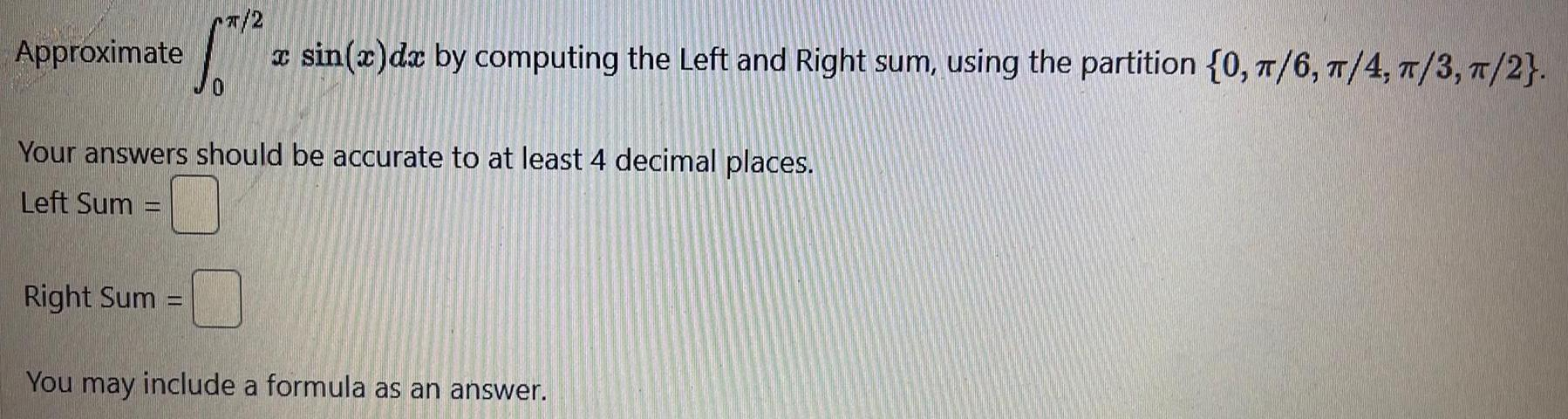Question:

# Approximate 2 x sin x dx by computing the Left and Right sum

Last updated: 1/23/2023Approximate 2 x sin x dx by computing the Left and Right sum using the partition 0 6 4 T 3 2 Your answers should be accurate to at least 4 decimal places Left Sum Right Sum You may include a formula as an answer